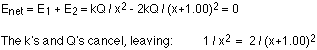## Friday, 3 October 2014

### CHARGES ARE IN EQUILIBRIUM EXAMPLE

Two charges are placed on the x axis. The first, with a charge of +Q, is at the origin. The second, with a charge of -2Q, is at x = 1.00 m. Where on the x axis is the electric field equal to zero?This question involves an important concept that we haven't discussed yet: the field from a collection of charges is simply the vector sum of the fields from the individual charges. To find the places where the field is zero, simply add the field from the first charge to that of the second charge and see where they cancel each other out.
In any problem like this it's helpful to come up with a rough estimate of where the point, or points, where the field is zero is/are. There is no such point between the two charges, because between them the field from the +Q charge points to the right and so does the field from the -2Q charge. To the right of the -2Q charge, the field from the +Q charge points right and the one from the -2Q charge points left. The field from the -2Q charge is always larger, though, because the charge is bigger and closer, so the fields can't cancel. To the left of the +Q charge, though, the fields can cancel. Let's say the point where they cancel is a distance x to the left of the +Q charge.Cross-multiplying and expanding the bracket gives:Solving for x using the quadratic equation gives: x = 2.41 m or x = -0.414 m
The answer to go with is x = 2.41 m. This corresponds to 2.41 m to the left of the +Q charge. The other point is between the charges. It corresponds to the point where the fields from the two charges have the same magnitude, but they both point in the same direction there so they don't cancel out.